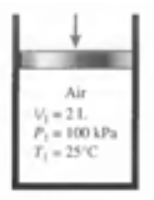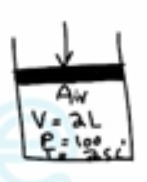Need Help?

Subscribe to Thermodynamics

###### \${selected_topic_name}
• Notes
• Comments & Questions

$\text { A piston-cylinder device initially contains } 2 \mathrm{L} \text { of air at } 100 \mathrm{kPa} \text { and } 25^{\circ} \mathrm{C} \text { . Air is now compressed }$

$\text { to a final state of } 600 \mathrm{kPa} \text { and } 150^{\circ} \mathrm{C} \text { . The useful work input is } 1.2 \mathrm{kJ} \text { . Assuming the }$

$\begin{array}{l}{\text { surroundings are at } 100 \mathrm{kPa} \text { and } 25^{\circ} \mathrm{C} \text { , determine (a) the exergy of the air at the initial }} \\ {\text { and the final states, (b) the minimum work that must be supplied to accomplish }} \\ {\text { this compression process, and (c) the second-law efficiency of this process. }}\end{array}$$A-1 \rightarrow R =0.287 kPa .m^3/kg.k$

$A - 2 \rightarrow C p=1.009 kJ/kg.k$

$C_ v=0.722 \mathrm{kJ} / \mathrm{kg} \cdot \mathrm{k}$

$m=\frac{P_1 V_{1}}{R T_{1}}=$$\frac{100 * 0.002}{0.287 * 298}=0.00234 \mathrm{kg}$

$\frac{P_2 V_2}{T_2}=\frac{P_1 V_1}{T_{1}} \longrightarrow V_{2}=\frac{P_{1} T_{2}}{P_{2} T_{1}} V_{1}$

$=\frac{100*423}{600*298}(2 L)=0.473 L$

$S_{2}-S_{0}=C_{p \ avrg} \ln \frac{T_{2}}{T_{0}}-R \ln \frac{P_2}{P_0}$

$=1.009*\ln \frac{423}{298}-0.287*\ln\frac{600}{100}=-0.1608 kJ/kg.k$

$X_{2}=\Phi_{2}=m([C_{v \ avrg}(T_2-T_0)-T_0(S_2-S_0)]+P_0(V_2-V_0))$

$=0.00234[(0.722 *(423-298)-298(-0.1608)+100*(0.000473-0.002)]$

$∴ {0.171} \mathrm{KJ}$

$X_{i n}-X_{ou t}-X_{destroied}=\Delta X_{system}$

$W_{rev, i n}=X_{2}-X_{1}$

$=0.171-0=0.171 k J$

$\eta_{II}=\frac{W_{rev \ in}}{W_{v, in}}=\frac{0.171}{1.2*100}=14.3 \%$

No comments yet